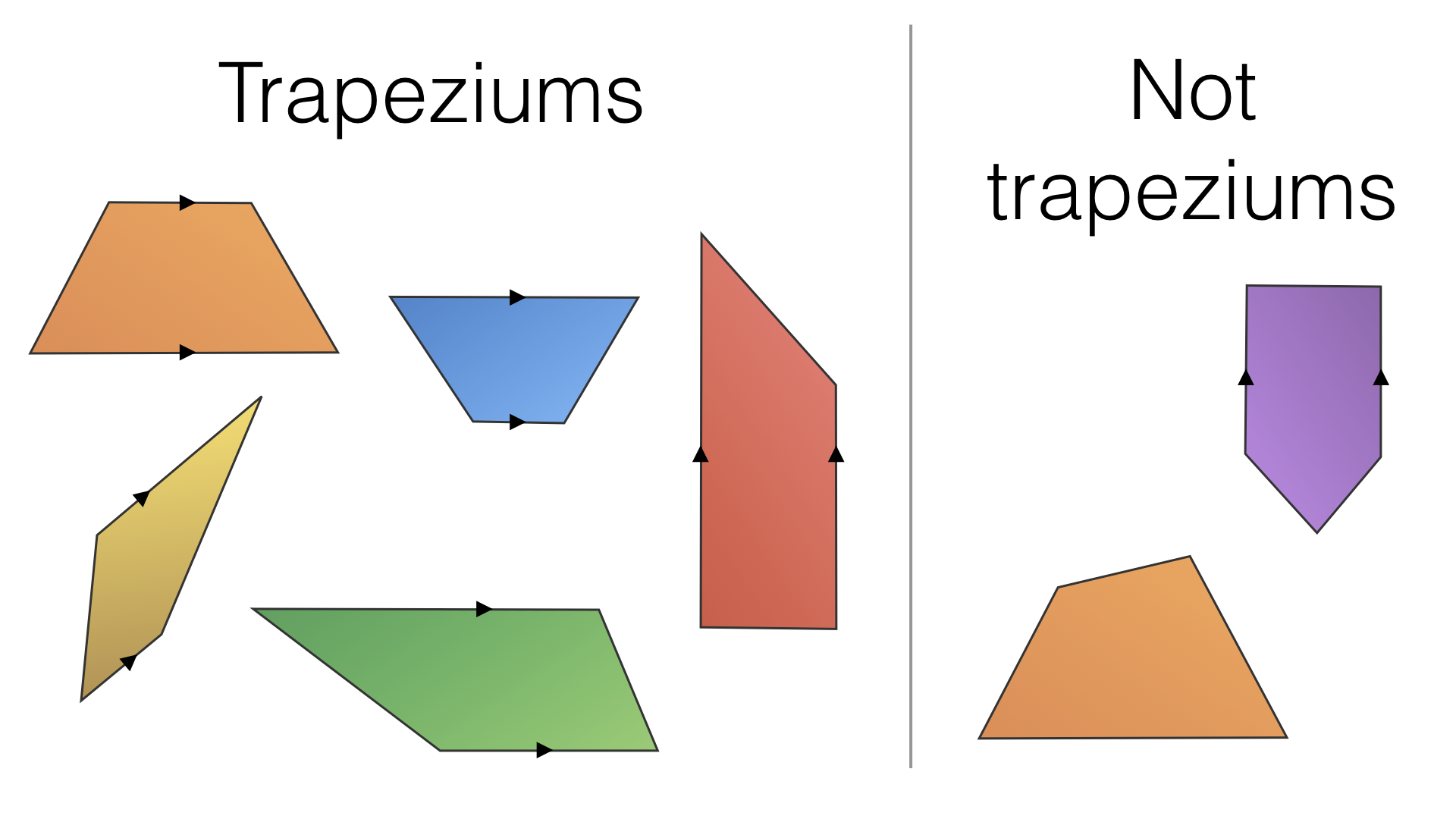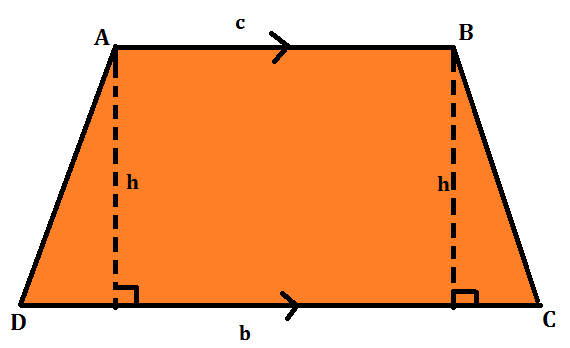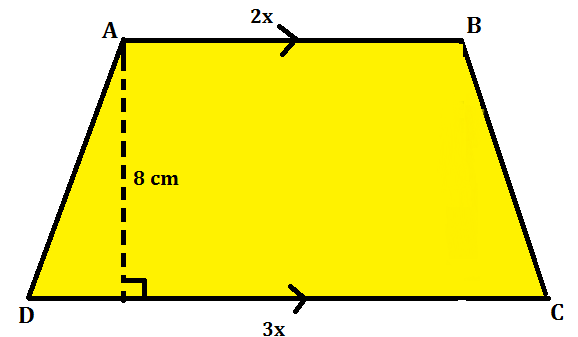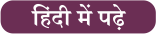Latest SSC jobs   »   Area Of Trapezium

# Area Of Trapezium: Definition, Properties, Formula And Examples

Area of Trapezium: Trapezium is a 2-dimensional geometric shape with 4 sides and 4 vertexes. It has at least one pair of parallel sides. in order to calculate the area of a trapezium, one must apply the appropriate formula to calculate the area of trapezium. This post comprises of the definition, properties, formula with examples of a trapezium to explain the students on the applications of the same.

## Area Of Trapezium: Definition

A trapezium is a 2D figure, itis a quadrilateral and has 4 sides out of which 2 sides are parallel to each other. The area of a trapezium is equal to the sum of the areas of the two triangles and the area of the rectangle. A trapezium where the two parallel sides are equal and form equal angles at one of the bases is called the isosceles trapezium.## Area Of Trapezium: Properties

Some of the properties of a trapezium are listed below:

1. The sum of the angles of a trapezium is 360º
2. A trapezium is not a parallelogram (as only one pair of opposite sides is parallel in a trapezium and we require both pairs to be parallel in a parallelogram).
3. The 4 sides of a trapezium are unequal unless it is an isosceles trapezium in which the 2 parallel sides are equal.
4. The diagonals of a trapezium bisect each other.
5. Two pairs of adjacent angles of a trapezium sum up to 180 degrees.

## The formula of the Area Of Trapezium

In order to calculate the area of a trapezium, you need to draw a perpendicular between the two parallel lines. The perpendicular will be donated as the height ‘h’ which is the distance between the parallel sides.Hence, the area of a trapezium is given by the formula:

## Area Of Trapezium Examples

Q1: The length of the two parallel sides of a trapezium are given in the ratio 3: 2 and the distance between them is 8 cm. If the area of trapezium is 400 cm², find the length of the parallel sides.

Solution:Let the 2 parallel sides as 3x and 2x.

Then, as the area of trapezium is 1/2 x distance between the parallel sides x Sum of parallel sides.

400= 1/2 x (3x + 2x) x 8

400 = 1/2 x 5x x 8

400 = 20x => x = 20 cm

The length of the parallel sides are 60 cm and 40 cm.

Q2. Two parallel sides of a trapezium are of lengths 27 cm and 19 cm respectively, and the distance between them is 14 cm. Find the area of the trapezium.

Solution:

Area of the trapezium = ¹/₂ × (sum of parallel sides) × (distance between them)

Area of the trapezium = {¹/₂ × (27 + 19) × 14} cm² = 322 cm²

Q3. The area of a trapezium is 352 cm² and the distance between its parallel sides is 16 cm. If one of the parallel sides is of length 25 cm, find the length of the other.

Solution:

Let the length of the required side be x cm.

Then, area of the trapezium = {¹/₂ × (25 + x) × 16} cm²

Area of the trapezium = (200 + 8x) cm².

But, the area of the trapezium = 352 cm² (given)

Therefore, 200 + 8x = 352

⇒ 8x = (352 – 200)

⇒ 8x = 152

⇒ x = (152/8)

⇒ x = 19.

The length of the other side is 19 cm.Sharing is caring!

June Current Affairs PDF

×

Thank You, Your details have been submitted we will get back to you.Join India's largest learning destination

What You Will get ?

•Job Alerts
•Daily Quizzes
•Subject-Wise Quizzes
•Current Affairs
•Previous year question papers
•Doubt Solving session

ORJoin India's largest learning destination

What You Will get ?

•Job Alerts
•Daily Quizzes
•Subject-Wise Quizzes
•Current Affairs
•Previous year question papers
•Doubt Solving session

ORJoin India's largest learning destination

What You Will get ?

•Job Alerts
•Daily Quizzes
•Subject-Wise Quizzes
•Current Affairs
•Previous year question papers
•Doubt Solving session

Enter the email address associated with your account, and we'll email you an OTP to verify it's you.Join India's largest learning destination

What You Will get ?

•Job Alerts
•Daily Quizzes
•Subject-Wise Quizzes
•Current Affairs
•Previous year question papers
•Doubt Solving session

Enter OTP

Please enter the OTP sent to
/6

Did not recive OTP?

Resend in 60sJoin India's largest learning destination

What You Will get ?

•Job Alerts
•Daily Quizzes
•Subject-Wise Quizzes
•Current Affairs
•Previous year question papers
•Doubt Solving sessionJoin India's largest learning destination

What You Will get ?

•Job Alerts
•Daily Quizzes
•Subject-Wise Quizzes
•Current Affairs
•Previous year question papers
•Doubt Solving session

Almost there

+91Join India's largest learning destination

What You Will get ?

•Job Alerts
•Daily Quizzes
•Subject-Wise Quizzes
•Current Affairs
•Previous year question papers
•Doubt Solving session

Enter OTP

Please enter the OTP sent to Edit Number

Did not recive OTP?

Resend 60

By skipping this step you will not recieve any free content avalaible on adda247, also you will miss onto notification and job alerts

Are you sure you want to skip this step?

By skipping this step you will not recieve any free content avalaible on adda247, also you will miss onto notification and job alerts

Are you sure you want to skip this step?